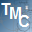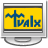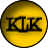# Math Calculator

2.6

Math Calculator is a program that enables you to calculate expressions, solve equations, find extremum, calculate derivatives and integrals. You can input an expression including variable x, for example, log(x), then input a value of x. You can also input an expression such as log(20) directly.

3.8
Rated by
82 users
Shareware \$20
OS:
Windows 7/XP
Date:
Category rank:
327
All software rank:
16 623

Become a user of Math Calculator v2.6, it won't take too long to download and set up the installer. The trial version of the GraphNow Software program gives access to most features, but to use it on the full you have to buy this program at \$20. The software can be found under the Science subcategory of the Education category. Among this tool versions, the most used ones are 2.5 and 2.3, which run under Windows 7/XP. The most popular installation files for Math Calculator are Math Calculator.exe and mathcal.exe.Download
Math Calculator 2.6

Thank you for rating the program!

### Useful softwareSmart Math Calculator
Define variables and solve mathematical equations and functions.